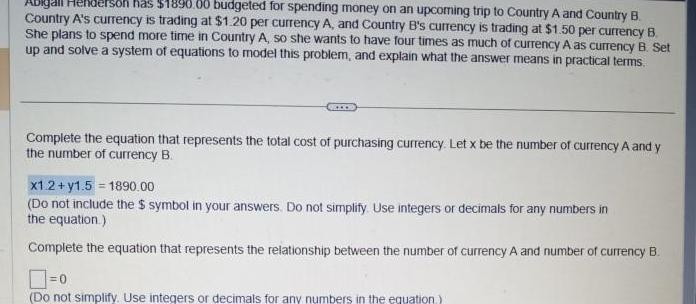Question:

# Abigall Henderson has 1890 00 budgeted for spending money on

Last updated: 3/20/2023Abigall Henderson has 1890 00 budgeted for spending money on an upcoming trip to Country A and Country B Country A s currency is trading at 1 20 per currency A and Country B s currency is trading at 1 50 per currency B She plans to spend more time in Country A so she wants to have four times as much of currency A as currency B Set up and solve a system of equations to model this problem and explain what the answer means in practical terms Complete the equation that represents the total cost of purchasing currency Let x be the number of currency A and y the number of currency B x1 2 y1 5 1890 00 Do not include the symbol in your answers Do not simplify Use integers or decimals for any numbers in the equation Complete the equation that represents the relationship between the number of currency A and number of currency B 0 Do not simplify Use integers or decimals for any numbers in the equation